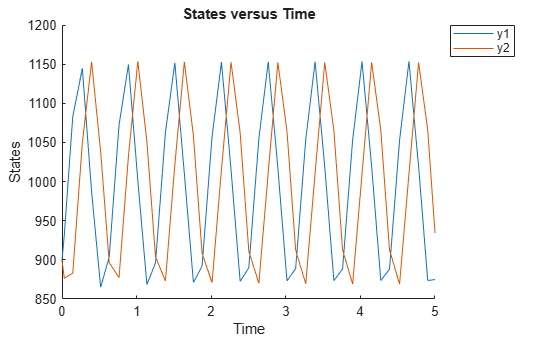# isAccelerated(SimFunction)

Determine if SimFunction object is accelerated

## Syntax

```tf = isaccelerated(F) tf = isaccelerated(F,computerType) ```

## Arguments

 `F` `SimFunction object` created by the `createSimFunction` method of a SimBiology model. `computerType` Character vector specifying a computer type. You can specify any valid `archstr` supported by the function `computer`.

## Description

`tf = isaccelerated(F)` returns true if `SimFunction object` `F` is accelerated for the current type of computer or `false` otherwise.

`tf = isaccelerated(F,computerType)` returns `true` if `F` is accelerated for the specified type of computer or `false` otherwise.

Note

`F` is automatically accelerated at the first function execution. However, manually accelerate the object if you want it accelerated in your deployment applications.

## Examples

collapse all

`sbioloadproject lotka;`

Create a SimFunction object f with c1 and c2 as input parameters to be scanned, and y1 and y2 as the output of the function with no dose.

`f = createSimFunction(m1,{'Reaction1.c1', 'Reaction2.c2'},{'y1', 'y2'}, [])`
```f = SimFunction Parameters: Name Value Type ________________ _____ _____________ {'Reaction1.c1'} 10 {'parameter'} {'Reaction2.c2'} 0.01 {'parameter'} Observables: Name Type ______ ___________ {'y1'} {'species'} {'y2'} {'species'} Dosed: None ```

The SimFunction object f is not set for acceleration at the time of creation. But it will be automatically accelerated when executed.

`f.isAccelerated`
```ans = logical 0 ```

Define an input matrix that contains parameter values for c1 and c2.

`phi = [10 0.01];`

Run simulations until the stop time is 5 and plot the simulation results.

`sbioplot(f(phi,5));`Confirm the SimFunction object f was accelerated during execution.

`f.isAccelerated`
```ans = logical 1 ```